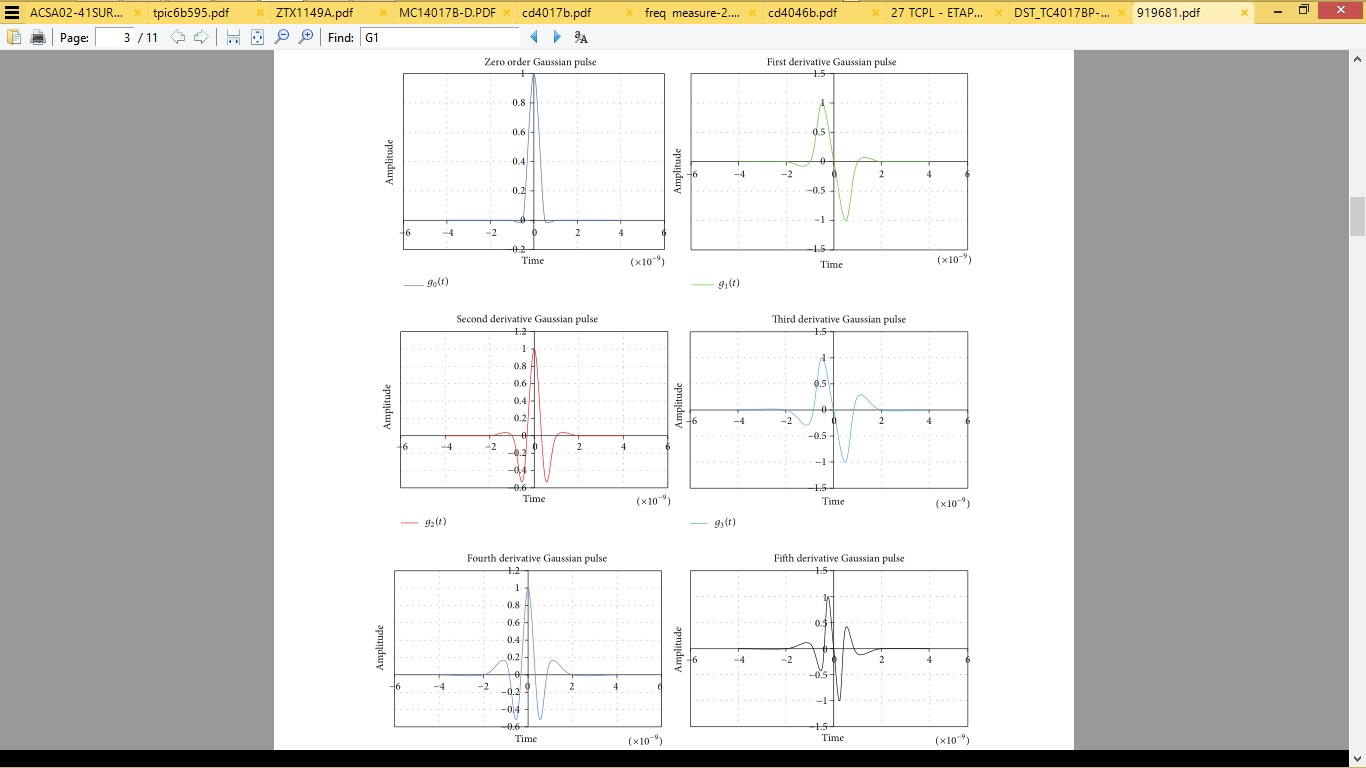# Energy in the signal

Status
Not open for further replies.

#### praveen450

##### Member level 5
Hello,

I'm implementing a matlab code to find energy in the gaussian signal. First I generated a gaussian signal and find the energy in it. Again I differentiated the signal 5 times and find the energy in it. Now, the energy in both the gaussian and 5th derivative gaussian signal should be same. But I'm unable to get this answer. Could anyone help me in this regard.
The matlab code is shown below :

Code ActionScript - [expand]1
2
3
4
5
6
7
8
9
10
11
12
13
14
15
16
17
18
19
20
clc
clear
b2=0
c=0.1e-9;
a2=1
x1=linspace(-1,1,2000);
x=x1.*10.^-8;
%%%%%%%%%%%%%%%%%%%%%%%%%%%%%%%%%%%%%%%%%%%%%%%%%% %%%%%%%%%%%%%%%%%%%%%%%%%%%%%%%%%
in1=exp(-((x)./c).^2);%zero-order gaussian signal
out=abs(in1.^2/50);%power of the signal
%energy =sum(out)%energy of the differentiated signal
energy=trapz(x,out)
%%%%%%%%%%%%%%%%%%%%%%%%%%%%%%%%%%%%%%%%%%%%%%%%%% %%%%%%%%%%%%%%%%%%%%%%%%%%%%%
%%%%%%%%%%%%%%%%%%%%%%%%%%%%%%%%%%%%%%%%%%%%%%%%%% %%%%%%%%%%%%%%%%%%%%%
%der2=-2*in1.*(c^2-2*x.^2)/(c^4);
der2=diff(in1,5)./(x(2)-x(1))^5;
der2=horzcat(der2,0,0,0,0,0)
out=abs(der2.^2/50);%power of the signal
%energy=sum(out)%energy of the differentiated signal
energy=trapz(x,out)

Last edited by a moderator:

On what basis do you believe it should be the same energy, if the lower portion of the spectrum is attenuated more than the upper.

I think the Gaussian shaped pulse when differentiated once it has a Guassian like spectrum. But 5 times?

Are you thinking of spectral density or pulse energy?

I'm considering pulse energy.
If you take a zero ordered gaussian signal, lets say it has energy E1 in time domain. Now if you differentiate n number of times integrate it over time, the differentiated signal should have same energy. Is it correct?.

if you differentiate n number of times integrate it over time, the differentiated signal should have same energy. Is it correct?.

Mathematically speaking, not: Integration operation adds a constant factor.
Anyway, differentiation at 5th order seems having no relation to any physical fundament.

I'm considering pulse energy.
If you take a zero ordered gaussian signal, lets say it has energy E1 in time domain. Now if you differentiate n number of times integrate it over time, the differentiated signal should have same energy. Is it correct?.

Any pulse, Gaussian or not has a finite DC energy unless it is AC coupled or differentiated causing undershoot on the trail edge where the area above and below zero are now equal. Differentiating the signal attenuates the spectrum 1/s or 6dB per octave or -6dB per harmonic, which changes the energy as repeating pulses have many harmonics up F=/PW50 pulse width then repeats with 2nd harmonics of the repetition rate,

Notice how the 2nd derivative looks like a QRS heart beat ... maybe integrated once. after trace show belowhttps://en.wikipedia.org/wiki/File:QRS_complex.pngStatus
Not open for further replies.